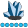Bal23
Lapis Lazuli | Level 10

## read the values, how to read the obs from the dataset

I need to read the values of the dependent variable y from the sas data I created into an n * K matrix, where n is the number of obs in each group and k is the number of levels of my cateogrial variable.   i need first to write k statements to read the obs from the dataset into k column vectors of dimension nX1, then needd to write a statment that horizontally concatenates these k columns vectors to form the n * k matrix

w

1 ACCEPTED SOLUTION

Accepted Solutions

## Re: read the values, how to read the obs from the dataset

If you look at my last message, you will see that there are no hard-coded values anywhere.  It should solve the problem as you have explained it.

13 REPLIES 13

## Re: read the values, how to read the obs from the dataset

You may need to supply some more details on how the SAS data set is structured to get better help.  However, my feeling is that you would be better to read the variable y into one vector, and then manipulate this is IML.  For example look at the SHAPE function which can be used to turn a vector into a matrix.Bal23
Lapis Lazuli | Level 10

## Re: read the values, how to read the obs from the dataset

a SAS data set that contains one continuous variable (y) and one categorical variable (x) with at least three levels. These are to be used as my continuous dependent variable and categorical independent variable in my single factor ANOVA model

then i will need to do sas iml

i have three groups, each has 11 obs

## Re: read the values, how to read the obs from the dataset

My suggestion to use SHAPE works as long as all the groups have the same size and the SAS data set is sorted by group.   Then you could use code like this:

``````  use mydata;
read all var {y} into y;
z = t( shape(y, 3, 11) );
print z;``````Bal23
Lapis Lazuli | Level 10

Thanks

## Re: read the values, how to read the obs from the dataset

or even simpler:

z = shapecol(y, 11);Bal23
Lapis Lazuli | Level 10

## Re: read the values, how to read the obs from the dataset

thanksBal23
Lapis Lazuli | Level 10

## Re: read the values, how to read the obs from the dataset

Then I realize I might need alternative code, that is, do not use 11, 3, but use a more general code, so that it can be fit for other similiar kind of dataset as well.

My question is, if others have simiar dataset, but instead of 11 obs, they have 20 obs, five categories, another dataset have 50 obs, four categories, can I have a more general code so that the code can be used for other datasets as well, "one size fits all"?

Thanks

## Re: read the values, how to read the obs from the dataset

You need to know either how many categories or how many obsevations.  You also need to make sure that each group has the same number of elements.

The best situation is if you have a categorical variable that tells you the group that each observations is associated with. Then you could do something like this

numGroups = ncol(unique(Group));

z = shapecol(y, 0, numGroups);

If you don't have a GROUP variable, you'll have to input the numGroups value manually.Bal23
Lapis Lazuli | Level 10

## Re: read the values, how to read the obs from the dataset

I do have a variable "Tem", it is a charactor not a numeric. It has three catogories

proc iml;

reset print;

use have;

numGroups = ncol(unique(tem));

z = shapecol(y, 0, numGroups);

ERROR: Y is not in the scope of variables for the data set.

does it mean that I have to convert the charactor to numeric variable?

## Re: read the values, how to read the obs from the dataset

No, the error is saying that you do not a variable named 'Y'.

Here is some data that shows you how it works:

``````data have;
do tem = 'A', 'B', 'C';
do i = 1 to 5;
y = rand("uniform");
output;
end;
end;

proc iml;
use have;
numGroups = ncol(unique(tem));
z = shapecol(y, 0, numGroups);
print z;``````

By the way, you mistakenly choose your own post as the solution. 🙂Bal23
Lapis Lazuli | Level 10

## Re: read the values, how to read the obs from the dataset

Thank you very much. This does show me how it works. . But my question remained unsolved, if I need a general code, rather than to type the number 3 and 11

## Re: read the values, how to read the obs from the dataset

If you look at my last message, you will see that there are no hard-coded values anywhere.  It should solve the problem as you have explained it.Bal23
Lapis Lazuli | Level 10

## Re: read the values, how to read the obs from the dataset

That is so good. Great learning experience! Thanks.

From The DO Loop